Function Repository Resource:

# GraphicsDirectiveQ

Test if an expression is a graphics directive

Contributed by: Enrique Zeleny
 ResourceFunction["GraphicsDirectiveQ"][expr] test if expr is a graphics directive.

## Details and Options

ResourceFunction["GraphicsDirectiveQ"] works for 2D and 3D directives.
Symbols that are considered to be graphics directives by ResourceFunction["GraphicsDirectiveQ"] are those mentioned on guide/GraphicsDirectives. As such, it may not support all directives.

## Examples

### Basic Examples (2)

Test common directives:

 In:=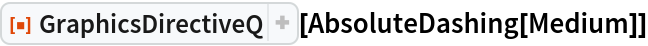Out=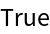In:=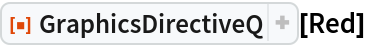Out=Test a more complicated directive:

 In:=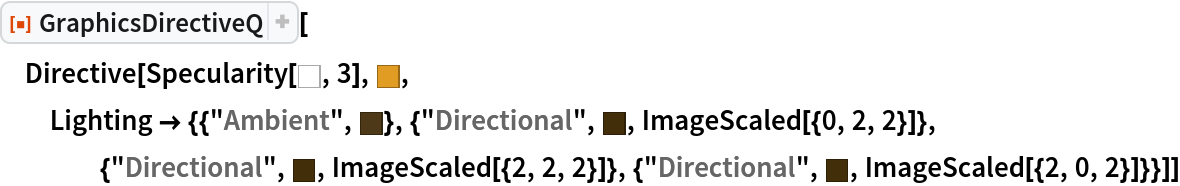Out=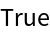### Scope (5)

A plot:

 In:=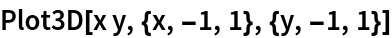Out=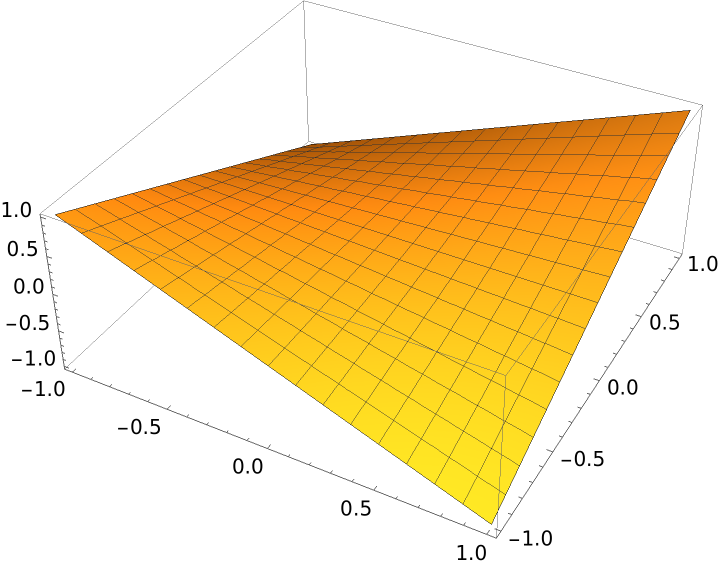Extract directives from the plot:

 In:=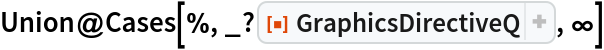Out=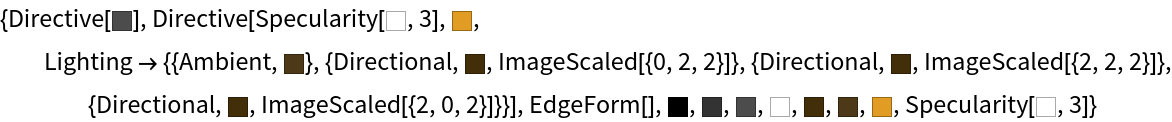The directive for a sphere with glow:

 In:=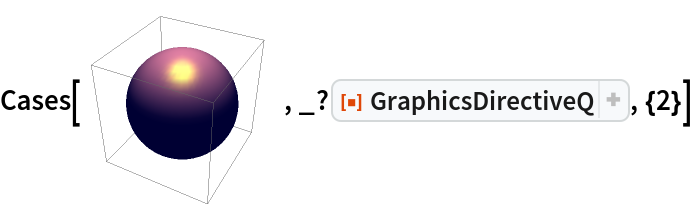Out=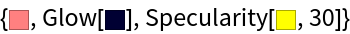Pass directives from the previous plot to another plot:

 In:=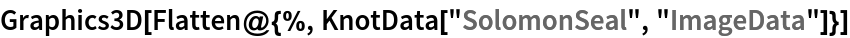Out=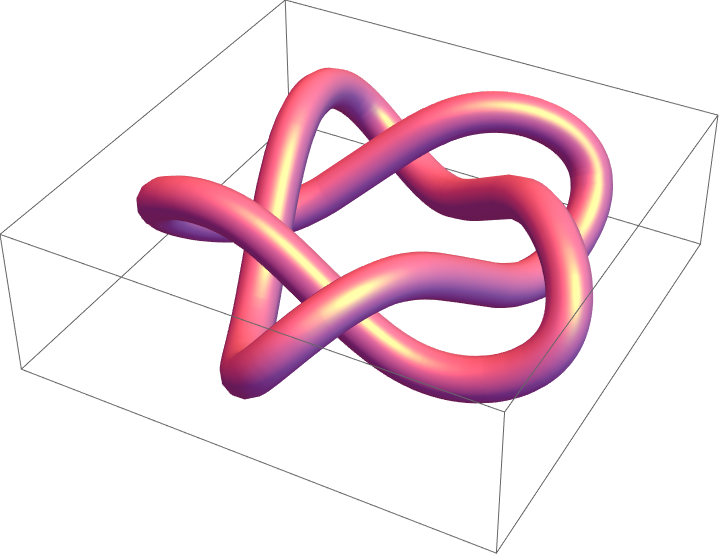Create a plot containing some directives:

 In:=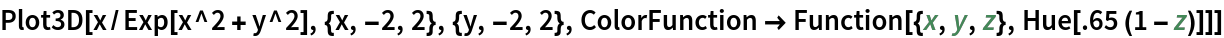Out=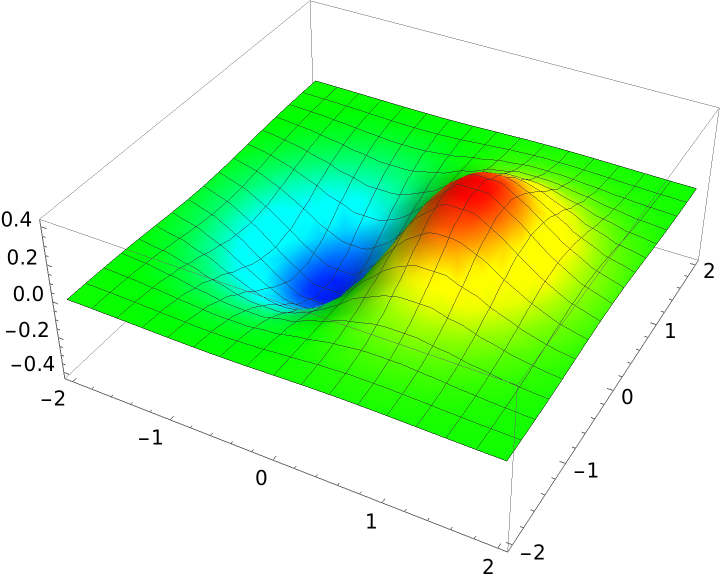Delete the directives within the plot:

 In:=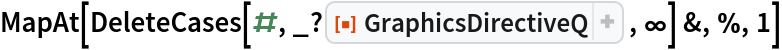Out=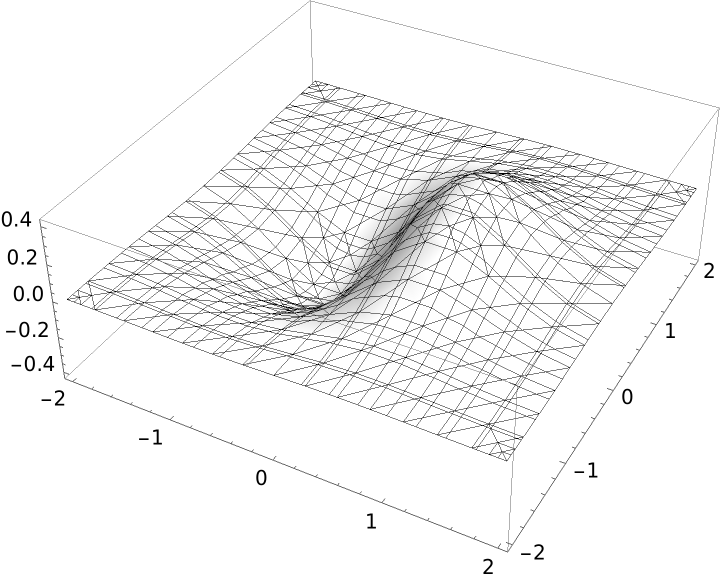Create a plot using a PlotTheme:

 In:=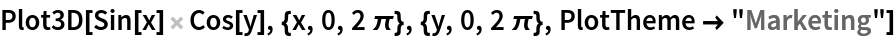Out=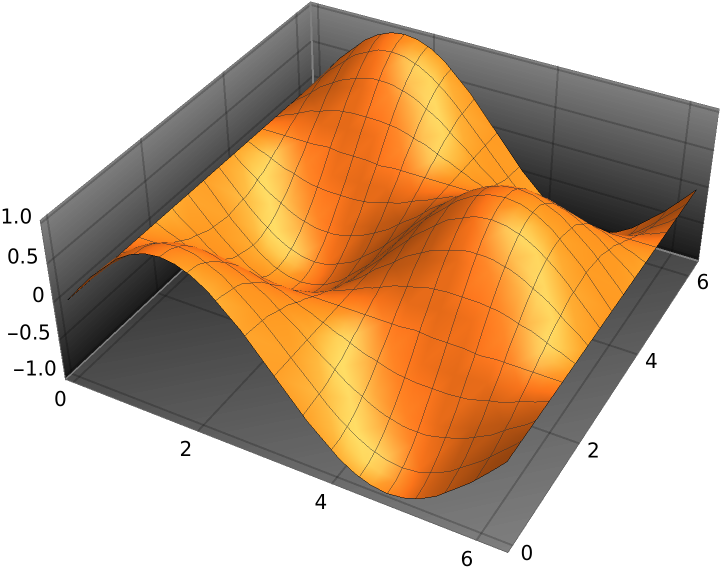Extract directives that appear in the previous output that were generated automatically by the PlotTheme:

 In:=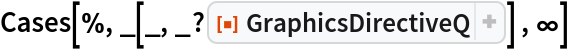Out=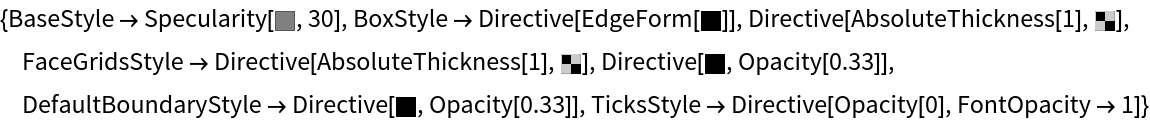Create a plot whose output contains some directives:

 In:=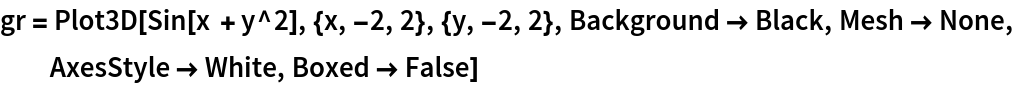Out=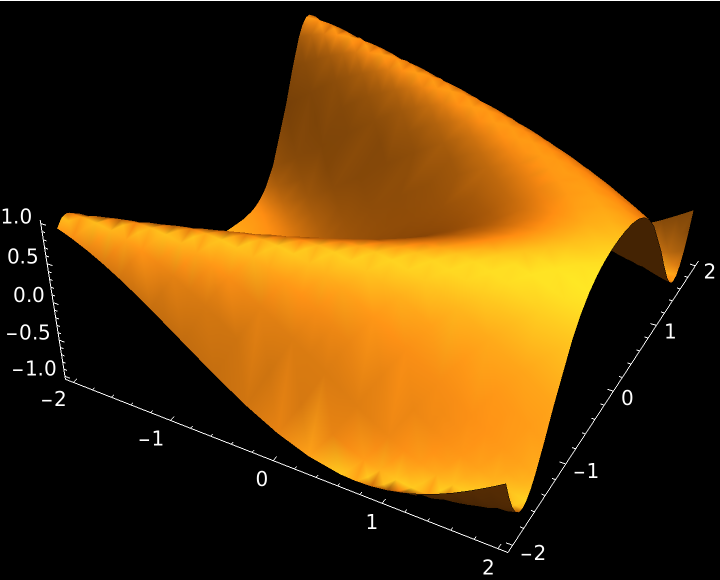Look at the structure of the code of the plot, showing explicitly only directives:

 In:=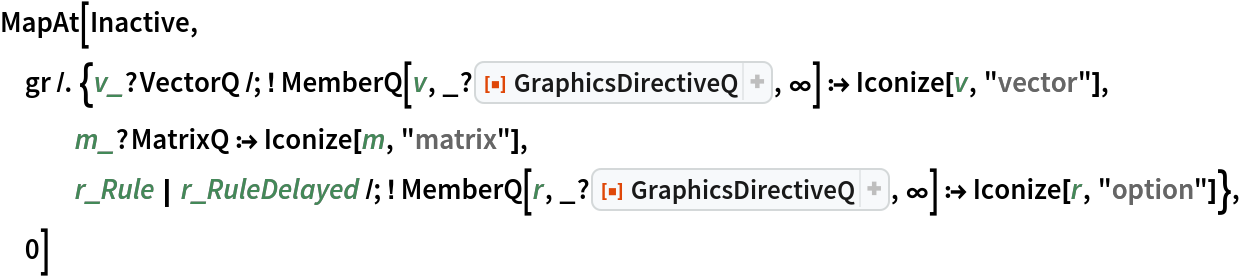Out=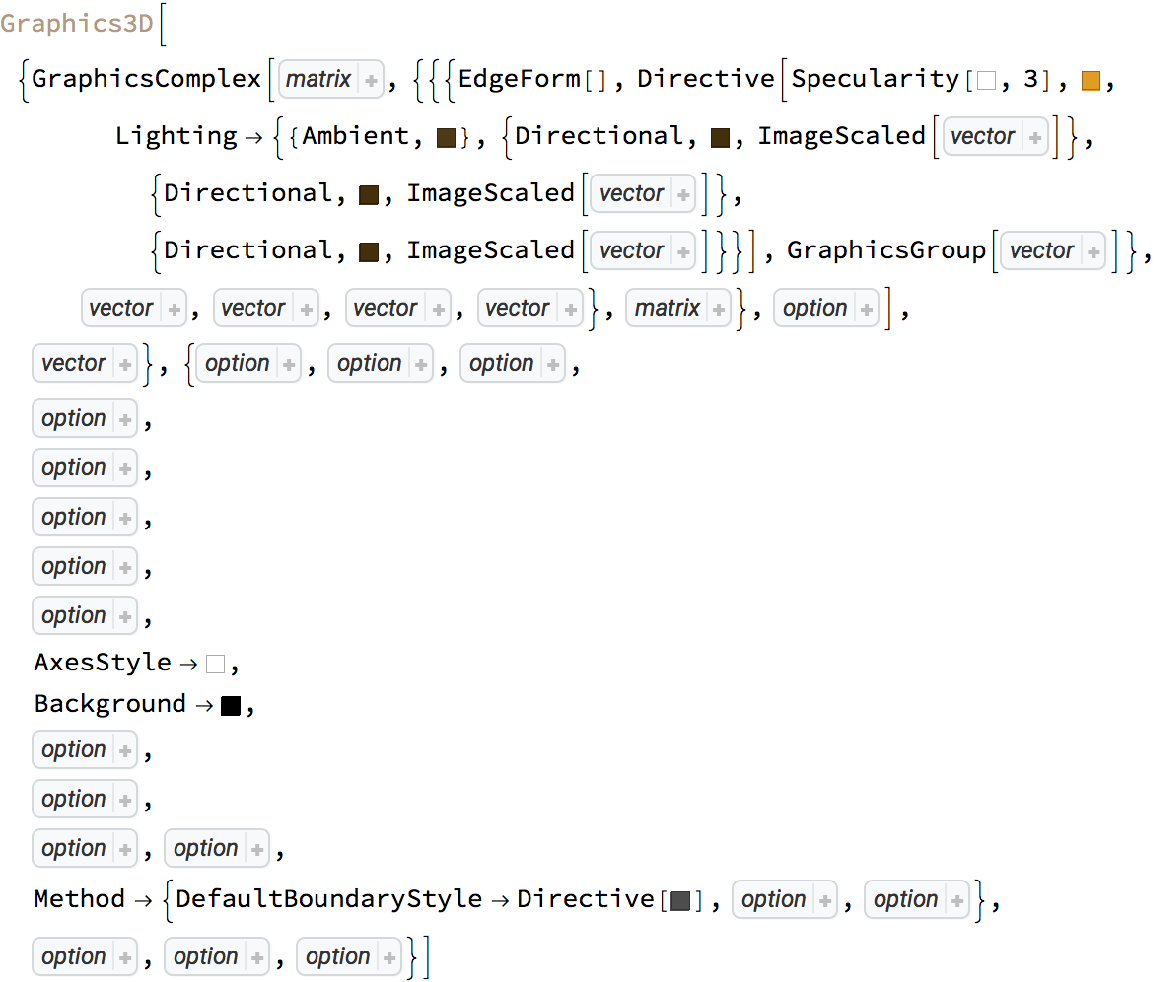Enrique Zeleny

## Version History

• 1.0.0 – 15 December 2020